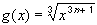#Interactive Real Analysis

Next | Previous | Glossary | Map

## 6.6. A Function Primer

### C(n) FunctionThe function g is n-times differentiable, the n-th derivative is continuous, and g is not (n+1)-times differentiable.

On the right are the graphs of this root function for n = 0, 1 and 2. Can you decide which graph goes with what n ? Click on Options and choose different powers of x to see how the function gets smoother and smoother at 0, which is necessary if it wants to be more and more times differentiable.

### Proof:

This proof is left as an exercise. As a hint, start with n = 1. The example, if correct, says that for n = 1 the function g is once differentiable, the derivative is continuous, but not differentiable again. Why ? Then try n = 2. Then, maybe, try an induction argument to prove the general case.
Next | Previous | Glossary | Map